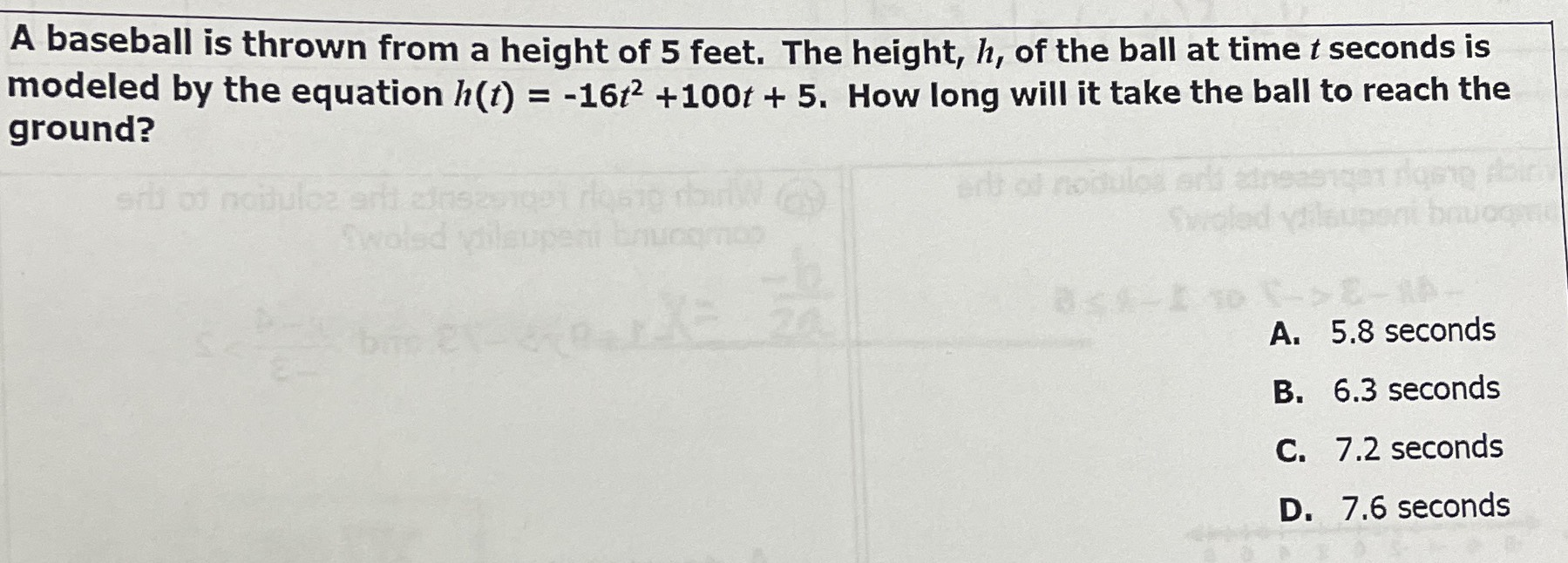### ¿Todavía tienes preguntas de matemáticas?

Pregunte a nuestros tutores expertos
Algebra
PreguntaA baseball is thrown from a height of $$5$$ feet. The height, $$h$$ , of the ball at time $$t$$ seconds is modeled by the equation $$h ( t ) = - 16 t ^ { 2 } + 100 t + 5$$ . How long will it take the ball to reach the ground?

A. $$5.8$$ seconds

B. $$6.3$$ seconds

C. $$7.2$$ seconds

D. $$7.6$$ seconds